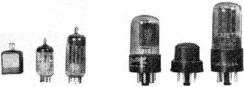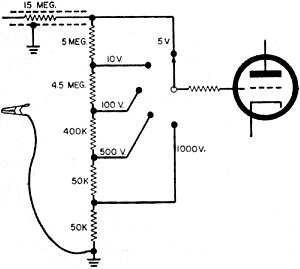# Test Instruments Part 4The Vacuum-Tube Voltmeter - D.C. RangesApril 1959 Popular Electronics

 April 1959 Popular ElectronicsTable of Contents Wax nostalgic about and learn from the history of early electronics. See articles from Popular Electronics, published October 1954 - April 1985. All copyrights are hereby acknowledged.
Popular Electronics ran a 5-part series on test equipment usage. This installment is on the use of a vacuum tube voltmeter (VTVM) for making DC measurements. Don't pass over the article just because it refers to a vacuum tube tester since there are lessons that apply to even the most modern transistorized, computerized meter. Author Larry Klein discusses mainly the DC functions, providing both functional descriptions of the circuits and how to use them for making accurate measurements. FET-input digital multimeters (DMMs) have largely replaced VTVMs, but they can still be found in some older electronics development labs and hobby benches.

Here is Part 5.

The Vacuum-Tube Voltmeter - D.C. Ranges

By Larry Klein
Technical EditorThe last three installments of this series were devoted to the volt-ohm-milliammeter. Now let's take a look at its chief competition - the vacuum-tube voltmeter (or VTVM).

Why put tubes in a voltmeter? To answer this question, it is necessary to understand the exact meaning of "sensitivity." As we found when checking out the VOM, the accuracy of anyFig. 1. Before measurement is taken, the voltage drops across the two 100,000 ohm resistors are equal. Shunting effect of meter causes an unequal voltage division and the meter reads less than half the voltage applied across A and C.
voltmeter reading depends upon the extent to which it affects the circuit under measurement. After the voltmeter is connected, the voltage at a particular point in a circuit frequently no longer has the value it had before the instrument was attached.

For example, with two equal-value resistors connected across a 100-volt source, the voltage across each resistor is 50 volts. (See Fig 1). However, a voltmeter connected across either resistor will read less than 50 volts. Why?

Well, if the VOM in Fig. 1 is a 1000-ohms/volt job, it has an internal resistance of 100,000 ohms (on the 100-volt range). Therefore, when the meter is connected, the total resistance between points Band C falls to 50,000 ohms. Since this is only half as great as the resistance between points A and B, the voltage divides unevenly and less voltage now appears between points B and C.Fig 2. Alternate versions of the VTVM bridge circuit. Their basic difference is mainly in the placement of the meter movement.
Of the total 100 volts, 66 2/3 volts will appear from A to B, and 33 1/3 volts will appear from B to C. The voltmeter therefore reads 33 1/3 volts even though 50 volts was present before the measurement was made.

The higher the internal resistance of a voltmeter, the less current it will drain from the circuit under test and the more accurate the reading will be. This is the advantage of the VTVM. Because of its very high input impedance, the VTVM provides a reading practically identical to the voltage existing before the test leads were connected.Zero adjust control balances the bridge circuit in the Precise Model 9071 VTVM.Range switch of the Eico Model 221 with precision divider resistors wired directly to the switch terminals for rigidity.Internal view of the Heathkit V7A VTVM. The range switch is on the left, function switch on the right.Four calibration controls of the Eico Model 221 are grouped around the meter movement as seen from the top of the chassis.The "active" elements in the VTVM. Standard models usually have a lineup comprising (from left to right) a 50-ma. power supply selenium rectifier, a 6AL5 a.c. rectifier and a 12AU7 bridge tube. An alternate arrangement consists of a 6X5 power rectifier, a 6H6 a.c. rectifier and a 6SN7 bridge tube.
Balanced Bridge. A check of the VTVM circuits shows that most current models use one or the other of the two bridge amplifiers in Fig.  2. In both circuits vacuum tube V1 serves as a d.c. bridge whose basic job is to effect an increase in the sensitivity of meter movement M1. Either circuit can also be considered as a means of decreasing the input current requirements for deflection of the meter-which comes down to the same thing. More on this point later.

Tube V1 is usually a 6SN7 or 12AU7 dual triode, and the indicating meter (M1) is connected from plate to plate or from cathode to cathode of the triodes. In both circuits, R1 adjusts for the normal differences between the operating currents of the two triodes and appears on the front panel of the VTVM as the Zero Adjust control.

Calibration control R2 is usually mounted inside the VTVM cabinet and adjusts for the small changes in total tube current over long periods of use.

If you're wondering why the VTVM circuits is referred to as a "bridge," as you can see Fig. 2 (B) can be easily redrawn to the standard bridge configuration (Fig. 3) and may even be more easily understood that way.

The theory of the bridge is quite simple. Triode V1b is the "reference" triode - in that its grid is grounded and the amount of current flowing through the tube is determined by the bias developed across cathode resistor R4 and the plate voltage of about 100 volts d.c. Triode V1a is operating under the same conditions except that its input grid is connected to a 5- or 6-resistor voltage divider which for the present discussion we will treat as a single resistor and call R5.

Zero Adjust control R1 varies the plate voltages to tube V1 in Fig. 2 (A) and varies the cathode bias in the circuit of Fig. 2 (B). By adjustment of R1, the voltage differences between the two plates or two cathodes can be reduced to zero - and the meter therefore will also read zero. We have a balanced condition.Fig. 3. The circuit of Fig. 2 (B) redrawn in the standard bridge configuration.Fig. 4. Voltage divider which sets the amount of the input voltage reaching the grid of the input triode.
Unbalancing the Bridge. Now that we have a stable balanced bridge, how do we go about making practical use of it? Referring back to Fig. 2, let's apply 1 volt d.c. between the input grid of V1a and ground and see what happens. When the applied positive voltage reduces the bias on V1a, the tube immediately reacts with an increase in current flow through R3. This, in turn, as Ohm's law tells us, causes an increased voltage drop across R3. Meter M1, up to this moment, has been comparing the drops across R3 and R4 and - not finding any difference - read "0." Now, though, M1 responds to the voltage difference between the plates or cathodes of V1, swings upscale - and we have a VTVM at work.

Let's take a look at R5. In a practical VTVM, R5 appears as the voltage divider shown in Fig. 4. The total voltage to be measured is always applied across the complete string of resistors in the input circuit.

For higher voltage ranges, the input grid of V1 is tapped farther down on the voltage divider circuit. Note that the input resistance remains constant and equals the total resistance of the voltage divider plus the isolating resistor in the d.c. probe. Adding up the resistances in the divider, you can see that the sensitivity of the VTVM is quite high.

The usual VTVM d.c. input resistance is 11 megohms and can be as high as 25 megohms. This is most important on the low voltage ranges of the VTVM which are often used for measurements in high impedance circuits. Note that, unlike the VOM, the input impedance of the VTVM remains constant regardless of which voltage range is used.

Next month we will continue our investigations and see what makes the VTVM able to respond to a million cycles a.c. and read up to a billion ohms resistance.

Posted  December 27, 2013About RF CafeCopyright: 1996 - 2024Webmaster:    Kirt Blattenberger,    BSEE - KB3UON RF Cafe began life in 1996 as "RF Tools" in an AOL screen name web space totaling 2 MB. Its primary purpose was to provide me with ready access to commonly needed formulas and reference material while performing my work as an RF system and circuit design engineer. The World Wide Web (Internet) was largely an unknown entity at the time and bandwidth was a scarce commodity. Dial-up modems blazed along at 14.4 kbps while typing up your telephone line, and a nice lady's voice announced "You've Got Mail" when a new message arrived... All trademarks, copyrights, patents, and other rights of ownership to images and text used on the RF Cafe website are hereby acknowledged. My Hobby Website:  AirplanesAndRockets.comPlease Support RF Cafe by purchasing my  ridiculously low−priced products, all of which I created. These Are Available for Free